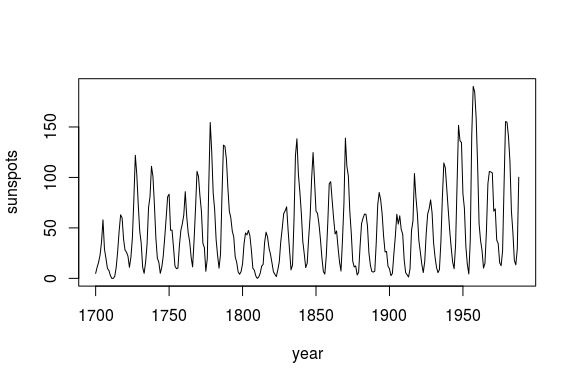rEDM

Overview

The rEDM package is a collection of methods for Empirical Dynamic Modeling (EDM). EDM is based on the mathematical theory of reconstructing attractor manifolds from time series data, with applications to forecasting, causal inference, and more. It is based on research software developed for the Sugihara Lab (University of California San Diego, Scripps Institution of Oceanography).

## Empirical Dynamic Modeling (EDM)

This package implements an R wrapper of EDM tools from the cppEDM library. Introduction and documentation are are avilable online, or in the package tutorial.

Functionality includes:

• Simplex projection (Sugihara and May 1990)
• Sequential Locally Weighted Global Linear Maps (S-map) (Sugihara 1994)
• Multivariate embeddings (Dixon et. al. 1999)
• Convergent cross mapping (Sugihara et. al. 2012)
• Multiview embedding (Ye and Sugihara 2016)

Installation

To install from CRAN:

install.packages(rEDM)

Using R devtools for latest development version:

install.packages("devtools")
devtools::install_github("SugiharaLab/rEDM")

Building from source:

git clone https://github.com/SugiharaLab/rEDM.git
cd rEDM
R CMD INSTALL .

Example

We begin by looking at annual time series of sunspots:

df = data.frame(yr = as.numeric(time(sunspot.year)),
sunspot_count = as.numeric(sunspot.year))

plot(df\$yr, df\$sunspot_count, type = "l",
xlab = "year", ylab = "sunspots")First, we use EmbedDimension() to determine the optimal embedding dimension, E:

# If you're new to the rEDM package, please consult the tutorial:
# vignette("rEDM-tutorial")

E.opt = EmbedDimension( dataFrame = df,    # input data
lib     = "1 280", # portion of data to train
pred    = "1 280", # portion of data to predict
columns = "sunspot_count",
target  = "sunspot_count" )E.opt
#     E    rho
# 1   1 0.7397
# 2   2 0.8930
# 3   3 0.9126
# 4   4 0.9133
# 5   5 0.9179
# 6   6 0.9146
# 7   7 0.9098
# 8   8 0.9065
# 9   9 0.8878
# 10 10 0.8773

Highest predictive skill is found between E = 3 and E = 6. Since we generally want a simpler model, if possible, we use E = 3 to forecast the last 1/3 of data based on training (attractor reconstruction) from the first 2/3.

simplex = Simplex( dataFrame = df,
lib     = "1   190", # portion of data to train
pred    = "191 287", # portion of data to predict
columns = "sunspot_count",
target  = "sunspot_count",
E       = 3 )

plot( df\$yr, df\$sunspot_count, type = "l", lwd = 2,
xlab = "year", ylab = "sunspots")
lines( simplex\$yr, simplex\$Predictions, col = "red", lwd = 2)
legend( 'topleft', legend = c( "Observed", "Predicted (year + 1)" ),
fill = c( 'black', 'red' ), bty = 'n', cex = 1.3 )Further Examples

Please see the package vignettes for more details:

browseVignettes("rEDM")

Sugihara G. and May R. 1990. Nonlinear forecasting as a way of distinguishing chaos from measurement error in time series. Nature, 344:734–741.

Sugihara G. 1994. Nonlinear forecasting for the classification of natural time series. Philosophical Transactions: Physical Sciences and Engineering, 348 (1688) : 477–495.

Dixon, P. A., M. Milicich, and G. Sugihara, 1999. Episodic fluctuations in larval supply. Science 283:1528–1530.

Sugihara G., May R., Ye H., Hsieh C., Deyle E., Fogarty M., Munch S., 2012. Detecting Causality in Complex Ecosystems. Science 338:496-500.

Ye H., and G. Sugihara, 2016. Information leverage in interconnected ecosystems: Overcoming the curse of dimensionality. Science 353:922–925.